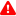* indicates a required field• phone number format invalid• email format invalidProblems detected, please review the form.
Privacy - Terms

# Curriculum Structure

Mathnasium's proprietary curriculum focuses on pinpointing each child's skills and knowledge to build a customized program designed to help them build their number sense — an intuitive understanding of numbers, their magnitude, relationships, and how they are affected by operations.## Counting

Counting is the ability to count from any number, to any number, by any number. It's the times tables (counting by 1, 2, 3 ... all the way to 12). Ultimately, it is the prediction, interpretation, and creation of patterns.

## Wholes & Parts

Knowledge of wholes and parts is the ability to “see” wholes and parts in a given question and to utilize the ideas “the whole equals the sum of its parts” and “each part equals the whole minus all of the other parts” to answer the question at hand. It's the basis for fractions, decimals, and all equations that are balanced parts of a whole.

## Proportional Thinking & Change

Proportional thinking and change is the ability to compare numbers by division and by subtraction and to use this knowledge to solve problems by “reasoning in groups.” Parts of the whole act in proportion to one another like the gears of a clock or geometric shapes coming together into a single form.

These three elements are further subdivided into the following 20 curricular areas:

• Counting
• Half
• Proportional Thinking
• Laws of Mathematics
• Fraction Concepts
• Percents
• Geometry
• Money
• Patterns
• Problem Solving
• Number Facts
• Computation
• Sameness, Quantity, Value
• Negative Numbers
• Number Theory
• Measurement
• Wholes and Parts
• Data Analysis
• Algebraic Thinking
• Math Vocabulary